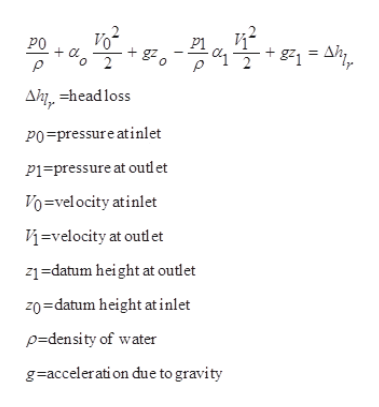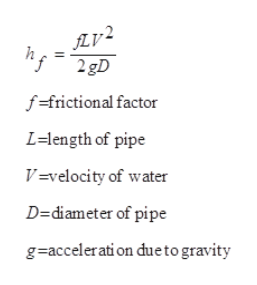# A cast-iron pipe system consists of a 500-ft section of water pipe, after which the flow branches into two 300-ft sections. The two branches then meet in a final 250-ft section. Minor losses may be neglected. All sections are 1.5-in nominal diameter, except for one of the two branches, which is 1- in nominal diameter. If the applied pressure difference is 100 psi, find the overall flow rate and the flow rate in each of the two branches. please attach the excel sheet and formula used

Question
2 views

A cast-iron pipe system consists of a 500-ft section of water pipe, after which the flow branches into two 300-ft sections. The two branches then meet in a final 250-ft section. Minor losses may be neglected. All sections are 1.5-in nominal diameter, except for one of the two branches, which is 1- in nominal diameter. If the applied pressure difference is 100 psi, find the overall flow rate and the flow rate in each of the two branches.

please attach the excel sheet and formula used

check_circle

Step 1

Applying bernoulli's equation,help_outlineImage TranscriptioncloseVo PO + g21 = Ah, Ahj, =headloss PO=pressure atinlet P1=pressure at outlet Vo=velocity atinlet Vý=velocity at outlet z1=datum height at outlet zo=datum height at inlet p=density of water g=acceleration due to gravity fullscreen
Step 2help_outlineImage TranscriptioncloseLV² 2 gD лг? f=frictional factor L=length of pipe V=velocity of water D=diameter of pipe g=accelerati on dueto gravity fullscreen
Step 3

calculatio...

### Want to see the full answer?

See Solution

#### Want to see this answer and more?

Solutions are written by subject experts who are available 24/7. Questions are typically answered within 1 hour.*

See Solution
*Response times may vary by subject and question.
Tagged in

### Fluid Mechanics